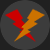Share via:

# AutoIt Random Function

The random function is used to generate random numbers.

1344 viewsEdited: 2017-12-19 16:44

The AutoIt Random function is used to generate a random number within a supplied range.

Random can be used in combination with sleep as a primitive anti-detection mechanism for certain automation tasks. Some systems might try to detect patterns in how a user behaves, and if a user is behaving too precise or repetitive, the user might be blocked or shown a CAPTCHA. Randomly sleeping might help to avoid those problems.

Random type functions can not truly be random, and therefor patterns might still arise over a very long time. However, this is not likely to cause problems with most detection measures.

Example:

```\$random_number = random(1, 10, 1) ; A value between 1 and 10 is generated
```

## Making random choices

You can also perform random actions using an if statement. To do this, you would usually make random return a value without decimals, so that you can more clearly write out your if checks. This is done by setting the flag parameter.

```\$random_number = random(1, 3, 1) ; A value between 1 and 3
```

Each number represents a unique action to be performed, so simply write an if statement for all numbers:

```\$random_number = random(1, 3, 1) ; A value between 1 and 3

If \$random_number = 1 Then
MsgBox(4096,"", "Value is 1.")
ElseIf \$random_number = 2 Then
MsgBox(4096,"", "Value is 2.")
Else
MsgBox(4096,"", "Value must be 3.")
EndIf
```

## Sleep a random amount of time

The below example shows how to use random together with the sleep function.

```\$st = random(1000, 5000, 1) ; Sleep Time

sleep(\$st) ; sleeps for a random amount of milliseconds between 1000 and 5000

MsgBox(0, "AutoIt Random Number Example:", \$st)
```

The next example shows the difference if you are using the integer flag.

```MsgBox(0, "AutoIt Random Number Example:", random(1000, 5000)) ; Outputs something like: 2524.55020649660

MsgBox(0, "AutoIt Random Number Example:", random(1000, 5000, 1)) ; Outputs something like: 4567
```

## Parameters

 Min [optional] The starting point, default is 0. Max [optional] The end point, default is 0. Flag [optional] The value of 1 returns an integer, while the default is a floating point number.